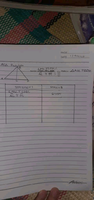# Congruence Of Triangle...Statement And Reason...ASA postulate.

#### Harry_the_cat

##### Senior Member
BC is a common side

#### Subhotosh Khan

##### Super Moderator
Staff member
Thank you, do you have another answer?
Please tell us how you used the information provided in response #2.

Are you familiar with theorem for "angle bisector of a triangle"?

#### Qwerty21

##### New member
Please tell us how you used the information provided in response #2.

Are you familiar with theorem for "angle bisector of a triangle"?
The supplementary, complementary? Like that? (sorry if my grammar is wrong)

#### Subhotosh Khan

##### Super Moderator
Staff member
The supplementary, complementary? Like that? (sorry if my grammar is wrong)

Are you familiar with theorem for "angle bisector of a triangle"?

If you are - please state it.

#### Qwerty21

##### New member

Are you familiar with theorem for "angle bisector of a triangle"?

If you are - please state it.
I searched it..

In geometry, the angle bisector theorem is concerned with the relative lengths of the two segments that a triangle's side is divided into by a line that bisects the opposite angle. It equates their relative lengths to the relative lengths of the other two sides of the triangle.

#### Harry_the_cat

##### Senior Member
OK. That's word for word from Wikipedia. But do you understand what it means and how you can apply it to your case?

#### Subhotosh Khan

##### Super Moderator
Staff member
I searched it..

In geometry, the angle bisector theorem is concerned with the relative lengths of the two segments that a triangle's side is divided into by a line that bisects the opposite angle. It equates their relative lengths to the relative lengths of the other two sides of the triangle.In your problem, the line BC is the bisector and it divides base AD into AB and BC. What do you deduce about ratio AB:BD?

You are given AC=CD. What does that lead to about AB:BD ?

#### Cubist

##### Full Member
The diagram doesn't seem to match with the vector expression $$\displaystyle \overline{AC}\equiv\overline{DC}$$ which implies that point A and D are exactly the same point.

#### Dr.Peterson

##### Elite Member
The diagram doesn't seem to match with the vector expression $$\displaystyle \overline{AC}\equiv\overline{DC}$$ which implies that point A and D are exactly the same point.
I don't think this is a vector problem. Those are line segments, not vectors; and they are congruent, not equal.

•Cubist

#### Dr.Peterson

##### Elite Member
I searched it..

In geometry, the angle bisector theorem is concerned with the relative lengths of the two segments that a triangle's side is divided into by a line that bisects the opposite angle. It equates their relative lengths to the relative lengths of the other two sides of the triangle.
I think you are telling us that you have not learned this theorem, but had to search for it. Therefore, it is not what you are expected to be using.

The supplementary, complementary? Like that? (sorry if my grammar is wrong)
This suggests that perhaps you observed that angles ACB and DCB are supplementary. That's a reasonable thing to think about, considering that the information you are given is SSA, and those angles would be interesting to work with. (It's possible that your work might replicate part of the proof of the angle bisector theorem!)

But the problem evidently is intended to use the ASA postulate. (Is that true?) Would you like to proceed using that, rather than the angle bisector theorem? If so, it will be very helpful if you show us what you have tried.